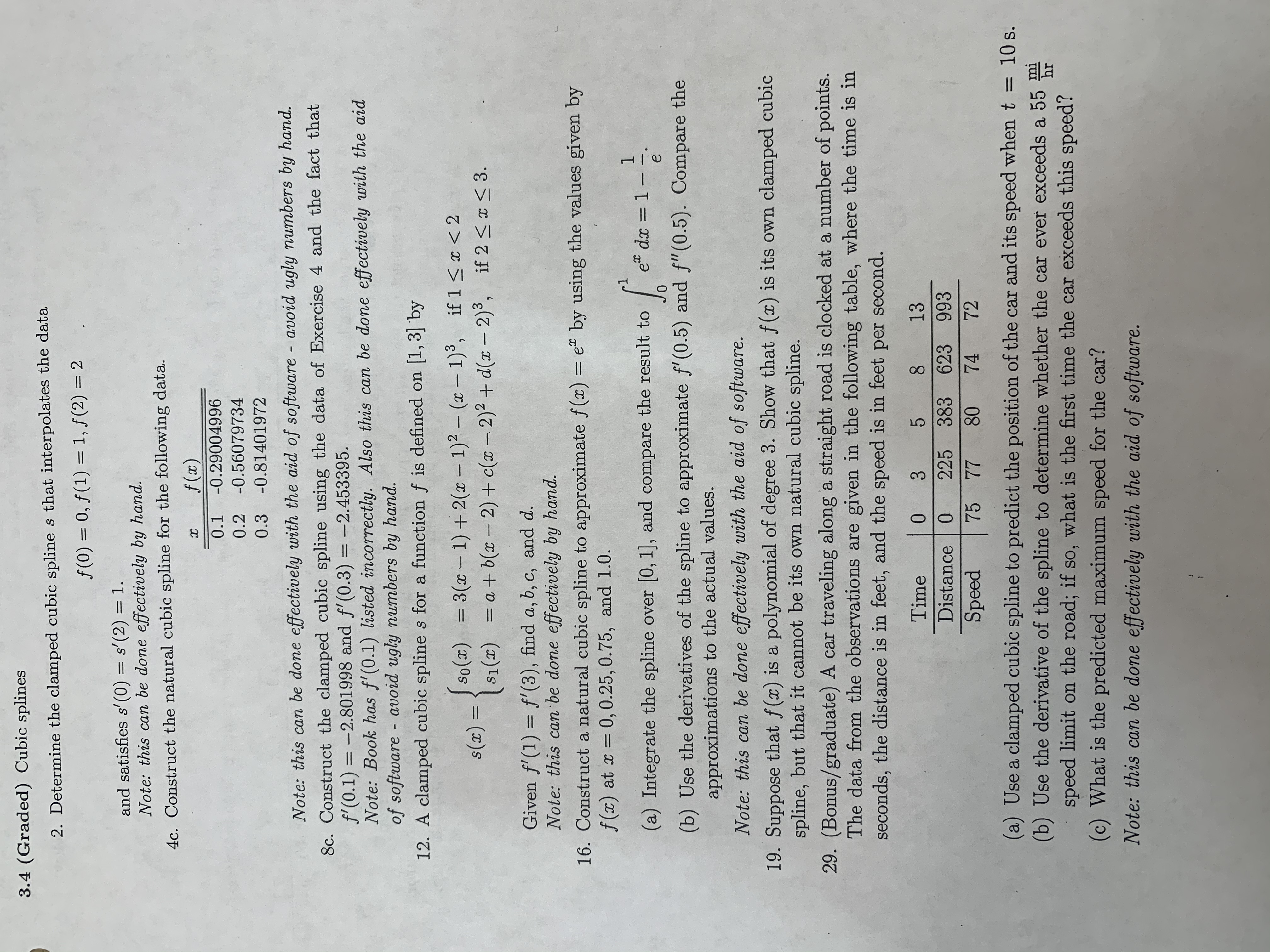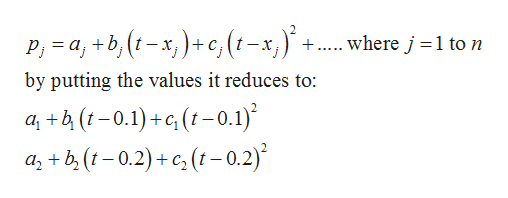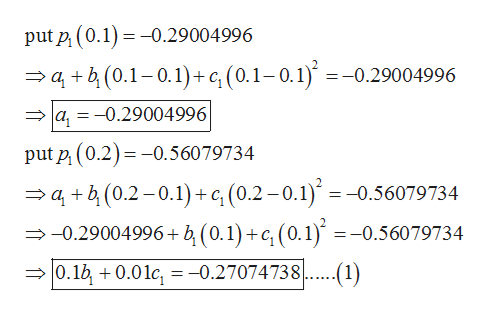# 3.4 (Graded) Cubic splines2. Determine the clamped cubic spline s that interpolates the dataf (0) 0, f(1) 1, f(2) 2and satisfies s' (0) = s'(2) = 1Note: this can be done effectively by hand.4c. Construct the natural cubic spline for the following data.f (x)х-0.290049960.1-0.560797340.2-0.814019720.3Note: this can be done effectively with the aid of software - avoid ugly numbers by hand.8c. Construct the clamped cubic spline using the data of Exercise 4 and the fact thatf'(0.1) =-2.801998 and f'(0.3) = -2.453395.Note: Book has f(0.1) listed incorrectly. Also this can be done effectively with the aidof software avoid ugly numbers by hand.12. A clamped cubic splines for a function f is defined on [1,3] byJso()3(-1)+2( -1)2 - (x - 1)3, if 1 < 2S1()a+b(x - 2)+ c( - 2)2 + d(x - 2)3,s(x) =if 2

Question
78 views

#4c from this handout

From Numerical Analysis 8th edition by Burden, Section 3.4help_outlineImage Transcriptionclose3.4 (Graded) Cubic splines 2. Determine the clamped cubic spline s that interpolates the data f (0) 0, f(1) 1, f(2) 2 and satisfies s' (0) = s'(2) = 1 Note: this can be done effectively by hand. 4c. Construct the natural cubic spline for the following data. f (x) х -0.29004996 0.1 -0.56079734 0.2 -0.81401972 0.3 Note: this can be done effectively with the aid of software - avoid ugly numbers by hand. 8c. Construct the clamped cubic spline using the data of Exercise 4 and the fact that f'(0.1) =-2.801998 and f'(0.3) = -2.453395. Note: Book has f(0.1) listed incorrectly. Also this can be done effectively with the aid of software avoid ugly numbers by hand. 12. A clamped cubic splines for a function f is defined on [1,3] by Jso()3(-1)+2( -1)2 - (x - 1)3, if 1 < 2 S1()a+b(x - 2)+ c( - 2)2 + d(x - 2)3, s(x) = if 2
check_circle

Step 1

From the given information:

Step 2

Here n+1=3 so, n=2

Now, define the polynomial pieces into piecewise polynomial as:help_outlineImage TranscriptioncloseP, a,b,(x)+c, (t-x,) where j 1 to n by putting the values it reduces to: a+(-0.1)+c(t-0.1) a+b(-0.2)+c(-0.2) fullscreen
Step 3

Now substitute the values to get...help_outlineImage Transcriptioncloseput p (0.1)0.29004996 ab(0.1-0.1)+ c, (0.1-0.1) =-0.29004996 a,-0.29004996 put p (0.2)0.56079734 ab(0.2-0.1)+c,(0.2-0.1) = -0.56079734 -0.29004996+ b (0.1)+c, (0.1) =-0.56079734 0.1b0.01c, = -0.27074738 fullscreen

### Want to see the full answer?

See Solution

#### Want to see this answer and more?

Solutions are written by subject experts who are available 24/7. Questions are typically answered within 1 hour.*

See Solution
*Response times may vary by subject and question.
Tagged in

### Math